# Brushed DC Motor Theory

(diff) ← Older revision | Latest revision (diff) | Newer revision → (diff)

## Introduction

The specific type of motor we are addressing is the permanent magnet brushed DC motor (PMDC). These motors have two terminals. Applying a voltage across the terminals results in a proportional speed of the output shaft in steady state.

There are two pieces to the motor: 1) stator and 2) rotor. The stator includes the housing, permanent magnets, and brushes. The rotor consists of the output shaft, windings and commutator. The image below shows a cut-away view of a Maxon motor. Note this picture has a gearbox and encoder attached to the motor.

## Motor Physics

The forces inside a motor that cause the rotor to rotate are called Lorentz Forces. If an electron is moving through a magnetic field, it experiences a force. If we have a current$\vec I$ passing through a wire in a magnetic field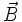$\vec B$, the wire experiences a force$\vec F$ proportional to the cross product of the current (expressed as a vector, including the direction of flow) and the magnetic field: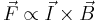$\vec F \propto \vec I \times \vec B\,$

You can easily find the direction of this force using the Right Hand Rule. The Right Hand Rule states that if you point your right hand's index finger along the direction of current, I, and your middle finger in the direction of magnetic flux, B, the direction of force is along the thumb. See the picture below.

Now, imagine this single wire is replaced with a loop of wire. Between the magnets' poles, this looks like two wires with current flowing in opposite directions. The forces on the wires cause the loop to rotate.

A coil consists of many such loops, and it is attached to the rotor and rotates. As it does so, the magnitude and direction of the forces on the wires remain approximately constant. However, the resultant torque varies with the angle. Look at the picture below. When the coil starts, there is maximum torque. As the coil moves, the moment arm is reduced and the torque decreases. Finally, when the coil is vertical (in the picture), there is no torque.

To keep a nearly constant torque on the rotor, we can do two things. First, we can reverse the current through the coil every half turn. So instead of an alternating torque like the one in the first figure below, the torque is always in the same direction. Also, additional coils can be used. When these coils are offset at different angles around the motor, the resultant torque becomes the sum of the colored torque curves in the figure below. The resultant torque is always greater than zero, but is not constant. This angle-dependent variation is called torque ripple.

The process of switching current direction is called commutation. To switch the direction of curent, brushed DC motors use brushes and commutators. The brushes are attached to the motor's two external wires, and the commutator segments slide over the brushes so that current through the coils switches at appropriate angles. Commutation can also be done electronically (see Brushless DC Motors). The following diagram shows how brushes and commutators work. A real commutator must have at least three segments.

## Equations

We start by writing the equation for conservation of energy in the motor. The power is input as electrical power,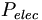$P_{elec}\,$ and the motor converts that to mechanical power,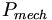$P_{mech}\,$. However, some of the power is lost as heat,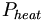$P_{heat}\,$, due to ohmic heating of the motor coils.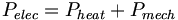$P_{elec}=P_{heat}+P_{mech}\,$

We can re-write this in terms of electrical and mechanical quantities as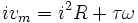$iv_m=i^2R+\tau\omega\,$

where vm is the voltage across the motor terminals, i is the current through the motor, τ is the torque produced by the motor, and ω is its angular velocity.

The wire coils have both a resistance, R, and an inductance, L. The motor's defining equation is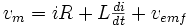$\begin{matrix}v_m = iR + L\frac{di}{dt} + v_{emf} \end{matrix}$

where vemf is called the back-emf (electromotive force). The back-emf is proportional to the speed of the motor,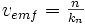$v_{emf} = \begin{matrix}\frac{n}{k_n}\end{matrix}\,$

where n is the rotation in revolutions-per-minute (rpm) (to be consistent with the Maxon datasheet below) and kn is called the speed constant, the inverse of which is often called the electrical constant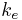$k_e\,$, which depends on the motor design. (My preference is to use SI units, using radians-per-second for angular velocity and volt-seconds/radians for the electrical constant and radians/volt-seconds for the speed constant. But speed constants are usually given in different units, and rpms are the most common angular velocity unit in motor data sheets.) The back-emf term indicates that any motor is also a generator: if we spin the motor shaft, we read a voltage at the motor terminals. This is how dams create hydroelectric power.

The torque generated by the motor is proportional to the current through the windings, where the constant of proportionality is called the torque constant kt or motor constant kM: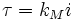$\tau = k_Mi\,$

The torque constant has the same numerical value as the inverse of the speed constant when they are expressed in the same SI units (use radians instead of revolutions, seconds instead of minutes, etc.), so there really is just one constant describing how our motor converts current to torque and angular velocity to voltage. To see this, remember the power equality ivm = i2R + τω, divide through by i, and you are left with vm = iR + kMω; in other words, kMω has taken the place of vemf = n / kn in the motor voltage equation (where di / dt = 0).

We are often interested in the steady-state operating characteristics of a motor, when the current i is constant. The term Ldi / dt becomes zero, and we get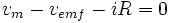$v_m-v_{emf}-iR=0\,$

which, using the relationships vemf = n / kn and τ = kMi, can be expressed equivalently as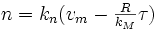$\begin{matrix} n = k_n (v_m - \frac{R}{k_M} \tau)\end{matrix}$

This equation allows us to plot the speed-torque curve of a motor. Given a constant vm, we can plot the speed of the motor as a function of the torque it produces (in steady state). The curve is just a straight line, as illustrated below. This equation allows us to calculate the stall (or starting) torque, by plugging in n = 0. This is the maximum torque the motor can generate. We can also calculate the stall current by dividing the stall torque by the motor constant (or, equivalently, by dividing vm by R). This is the maximum current the motor will draw. We can also calculate the no-load speed, the maximum speed the motor will achieve, by assuming it provides zero torque (i.e., there is no friction resisting motion) and plugging in τ = 0. See the example motor datasheet below.

## Datasheets and Speed-Torque Curves

The datasheet below is for the Maxon motor available in the Mechatronics Lab. That motor also has an integrated 6:1 gearhead and a 100 line encoder. The motor is rated at 6 W, which is approximately the input power (voltage times current) when the motor is operated at the nominal voltage (24 V) and maximum continuous torque, where the current is approximately 240 mA.

1. Nominal voltage: The recommended maximum voltage across the motor terminals, and the voltage for which the speed-torque curve (below) is plotted. The motor can be powered with less or more voltage, but higher voltage should be used with care, to prevent the motor coils from overheating due to ohmic (resistive) heating.

2. No load speed,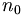$n_0\,$: The speed of the motor powered by the nominal voltage when the motor provides zero torque.

3. No load current: The current required to spin the motor at the no load condition (i.e., the current needed to provide the torque necessary to overcome friction).

4. Nominal speed: The speed of the motor at the maximum continuous torque.

5. Nominal torque (max continuous torque): The maximum torque the motor can provide continuously without overheating.

6. Nominal current (max continuous current): The current that yields the maximum continuous torque. This maximum is determined by thermal characteristics of the motor. The power dissipated by the motor as heat is i2R. Larger currents are acceptable intermittently, but large continuous currents may cause the motor to overheat.

7. Stall torque,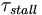$\tau_{stall}\,$: The maximum torque achievable by the motor at the nominal voltage. This torque is achieved at zero velocity (stall).

8. Starting current,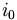$i_0\,$: The current through the motor at zero velocity, equal to the nominal voltage divided by the terminal resistance. Also called the stall current.

9. Max efficiency: The maximum efficiency of the motor in converting electrical power to mechanical power. This maximum efficiency typically occurs at high speed and low torque; the efficiency is zero at zero speed and zero torque, since the mechanical power is τω.

10. Terminal resistance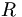$R\,$: The resistance of the motor windings.

11. Terminal inductance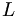$L\,$: The inductance of the motor windings.

12. Torque (or motor) constant,$k_t\,$ or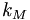$k_M\,$: The constant of proportionality relating current to torque. In SI units (Newton-meters per amp), the torque constant is equivalent to the inverse of the speed constant in SI units (radians per volt-second).

13. Speed constant,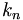$k_n\,$ (which is the inverse of the electrical constant$k_e\,$): The constant of proportionality relating speed to voltage. Equivalent to the inverse of the torque constant when expressed in SI units.

14. Speed/torque gradient: A representation of the slope of the speed-torque curve (see graph below), approximately equal to the no load speed divided by the stall torque.

15. Mechanical time constant: The time it takes the unloaded motor to reach 63% of its no load speed under a constant voltage, starting from rest. Proportional to the inertia of the rotor and inversely proportional to the square of the the torque constant.

16. Rotor inertia: The inertia of the rotating element (the rotor) about the axis of rotation.

Much of the data sheet can be expressed in the speed-torque curve, plotted for the constant nominal voltage (below). The mechanical power output is the product of the torque and the speed, and is maximized at half the maximum speed and torque.

Often you need higher torques but lower speeds in your application. In this case, you can add a gearbox to the motor, as discussed in Choosing a Motor and Gearing Combination.

## Other Effects

• Cogging. With no power to the motor, you'll feel the motor shaft bump-bump-bump as you spin it by hand, due to the orientation-dependent attraction of the rotor to the permanent magnets.
• Friction losses.